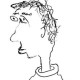# Artin vanishing is false in rigid geometry

Sorry for the lack of blogging.  It’s been a busy semester.

Let$k$ be an algebraically closed field, and let$X$ be a$d$-dimensional affine variety over$k$.  According to a famous theorem of Artin (Corollaire XIV.3.5 in SGA 4 vol. 3), the etale cohomology groups$H^i_{\mathrm{et}}(X,G)$ vanish for any$i > d$ and any torsion abelian sheaf$G$ on$X_{\mathrm{et}}$. This is a pretty useful result.

It’s natural to ask if there’s an analogous result in rigid geometry.  More precisely, fix a complete algebraically closed extension$k / \mathbf{Q}_p$ and a$d$-dimensional affinoid rigid space$X=\mathrm{Spa}(A,A^\circ)$ over$k$.  Is it true that$H^i_{\mathrm{et}}(X,G)$ vanishes for (say) any$i>d$ and any$\mathbf{Z}/n\mathbf{Z}$-sheaf$G$ on$X_{\mathrm{et}}$ for$n$ prime to$p$?

I spent some time trying to prove this before realizing that it fails quite badly.  Indeed, there are already counterexamples in the case where$X=\mathrm{Spa}(k \langle T_1,\dots,T_d \rangle,k^\circ \langle T_1, \dots, T_d \rangle)$ is the$d$-variable affinoid disk over$k$.  To make a counterexample in this case, let$Y$ be the interior of the (closed, in the adic world) subset of$X$ defined by the inequalities$|T_i| < |p|$ for all$i$; more colloquially,$Y$ is just the adic space associated to the open subdisk of (poly)radius$1/p$. Let$j: Y \to X$ be the natural inclusion.  I claim that$G = j_! \mathbf{Z}/n\mathbf{Z}$ is then a counterexample.  This follows from the fact that$H^i_{\mathrm{et}}(X,G)$ is naturally isomorphic to$H^i_{\mathrm{et},c}(Y,\mathbf{Z}/n\mathbf{Z})$, together with the nonvanishing of the latter group in degree$i = 2d$.

Note that although I formulated this in the language of adic spaces, the sheaf$G$ is overconvergent, and so this example descends to the Berkovich world thanks to the material in Chapter 8 of Huber’s book.

It does seem possible, though, that Artin vanishing might hold in the rigid world if we restrict our attention to sheaves which are Zariski-constructible.  As some (very) weak evidence in this direction, I managed to check that$H^2_{\mathrm{et}}(X,\mathbf{Z}/n \mathbf{Z})$ vanishes for any one-dimensional affinoid rigid space$X$.  (This is presumably well-known to experts.)

## 2 thoughts on “Artin vanishing is false in rigid geometry”

1.none says:

Does this mean that there is no rigid analogue of perverse sheaves?

Like

1.arithmetica says:

No naive analogue, at least.

Like